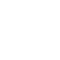# Introduction to Electrostatic Sensors and Actuators

A capacitor is broadly defined as two conductors that can hold opposite charges. It can be used as either a sensor or an actuator. If the distance and relative position between two conductors change as a result of applied stimulus, the capacitance value would be changed. This forms the basis of capacitive (or electrostatic) sensing.

On the other hand, if a voltage (or electric field) were applied across two conductors, an electrostatic force would develop between these two objects. This is defined as electrostatic actuation.

Electrostatic forces are not often used for driving macroscopic machinery. However, micro devices have large surface-area-to-volume ratios and their masses are generally very small, thus making electrostatic force, which is a surface force, an attractive candidate for micro actuation.The electrostatically driven micro motor was one of the earliest MEMS actuators. The motor, schematically diagrammed in figure, consists of a rotor that is attached to the substrate with a hub, and a set of fixed electrodes on the periphery, called stators.

The stators are grouped together such that each group of four electrodes is electrically biased simultaneously. Three such groups are present for the motor shown in figure and are identified by different fill patterns.

To explain the operation principle of the electrostatic motor, let’s begin with the rotor at an arbitrary angular rest position (Figure a).

One group of stator electrode is first biased. (The electrodes that are biased at a particular stage are identified by arrows placed next to them.)

An in-plane electric field develops between any given stator electrode in this group and the closest rotor tooth next to it. This generates an electrostatic attractive force that aligns the said tooth with the said stator electrode. The torque values are on the order of pico Nm for voltages of the order of 100 V, large enough to overcome static friction. A small angular movement of the rotor is made to reach a new configuration diagramed in figure b.

The electric bias is shifted to the next group of stator electrodes (Figure c), resulting in another small angular movement in the same direction. Continuous motion of the rotor can be achieved by activating the stator electrodes by groups in succession.

Simplicity – The sensing and actuation principles are relatively easy to implement, requiring only two conducting surfaces. No special functional materials are required.

Other sensing methods, such as piezoresistive and piezoelectric sensing, and other actuation methods, such as piezoelectric actuation, require deposition, patterning, and integration of special piezoresistive and piezoelectric materials.

Low power – Electrostatic actuation relies on differential voltage rather than current. The method is generally considered energy efficient for low-frequency applications.

This is especially true under static conditions, when no current is involved. At high frequencies, a time-and frequency-dependant displacement current, i(t), will develop in response to a time varying bias voltage V(t).

The magnitude of the current is $i(t)=C \frac{d V(t)}{d t}$

The instantaneous power delivered to a capacitor is p(t) = i(t) . V(t).

Fast response – Electrostatic sensing and actuation offers high dynamic response speed, as the transition speed is governed by the charging and discharging time constants that are typically small for good conductors. For example, the switching time of the mirrors in the digital micromirror display (DMD) array is smaller than 21 μs, fast enough to support 8-bit gray scale display.

Relative disadvantages of electrostatic actuation and sensing should be recognized as well. High voltage required for static actuator operation is considered a drawback.

The DMD mirrors are switched by voltage on the order of 25 V to achieve ±7.5 degree tilting. A monolithically integrated optical mirror with 9° tilt range requires 150 V bias voltage.

Linear electrostatic actuators requiring hundreds of volts to move a microstructure by several tens of micrometers are rather common. High voltage introduces electronics complexity (for providing high voltage supply) and material compatibility issues.

Electrodes that are mechanically connected with insulators tend to accumulate charges, especially under DC operation modes. The trapped charges will change the operation characteristics.